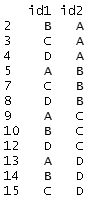## Monday, February 6, 2012

### Using apply() to create a unique id

Suppose you have a data set with two identifiers. For example, maybe you're studying the relationships among firms in an industry and you have a way to link the firms to one another. Each firm has an id, but the unique unit in your data set is a pairing of ids. Here's a stylized example of one such data set:In the example that motivated this post, I only cared that A was linked with B in my data, and if B is linked with A, that's great, but it does not make A and B any more related. In other words, the order of the link didn't matter.

In this case, you'll see that our stylized example has duplicates -- id1 = "A" and id2 = "B" is the same as id1="B" and id2 = "A" for this purpose. What's a simple way to get a unique identifier? There's an apply command for that!

Thinking of each row of the identifier data as a vector, we could alphabetize (using sort(), so c("B", "A") becomes c("A", "B")), and then paste the the resulting vector together into one identifier (paste, using collapse). I call our worker function idmaker():
idmaker = function(vec){
return(paste(sort(vec), collapse=""))
}
Then, all we need to do is use the apply command to apply this function to the rows of the data, returning a vector of results. Here's how my output looks.To get a data frame of unique links, all we need to do is cbind() the resulting vector of indices to the original data frame (and strip the duplicates). Here's some code:

co_id = apply(as.matrix(df[, c("id1", "id2")]), 1, idmaker)
df = cbind(df, co_id)
df = df[!duplicated(df[,"co_id"]),]

Here is the resulting data frame with only unique pairs.